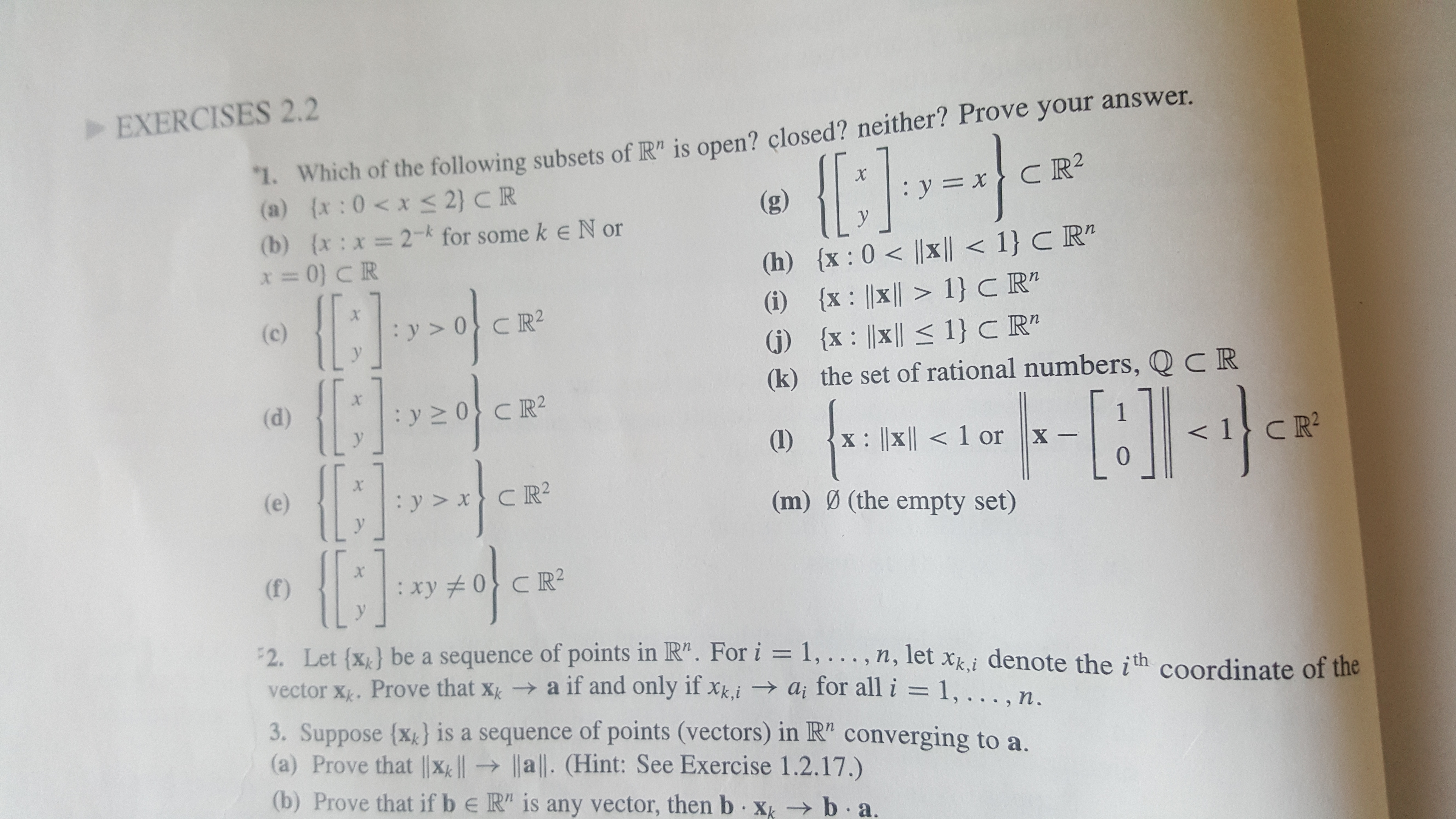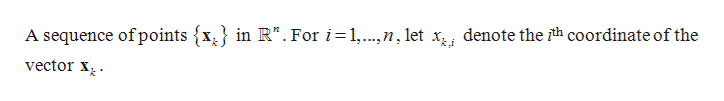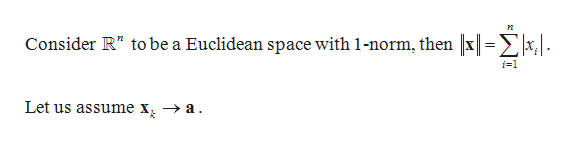# EXERCISES 2.2"1. Which of the following subsets of R" is open? closed? neither? Prove your answer.(a) (x :0 x< 2} CR(b) (x:x= 2 for some k eN or=0) C RXCR2y=x(g)y(h) {x: 0 |x|| < 1} C R"(i) x |x 1} C R"(G) x ||xl1} C R"(k) the set of rational numbers, Q C RXr(c)X-(d)1X-10(1I)X: |x1 orC R2(e)CR2(m) Ø (the empty set)X(f)xy 0 R2y2. Let {x&} be a sequence of points in R". For i = 1, .. . , n, let xki denote the ith coordinate of thevector x. Prove that xk a if and only if xkiai for all i = 1, ....n3. Suppose (x} is a sequence of points (vectors) in R" converging to a.(a) Prove that |x all. (Hint: See Exercise 1.2.17.)(b) Prove that if b e R" is any vector, then b xk b a.

Question
1 views

I need help for the proof of question 2. Thank you very much!help_outlineImage TranscriptioncloseEXERCISES 2.2 "1. Which of the following subsets of R" is open? closed? neither? Prove your answer. (a) (x :0 x< 2} CR (b) (x:x= 2 for some k eN or =0) C R X CR2 y=x (g) y (h) {x: 0 |x|| < 1} C R" (i) x |x 1} C R" (G) x ||xl1} C R" (k) the set of rational numbers, Q C R Xr (c) X- (d) 1 X- 10 (1I) X: |x 1 or C R2 (e) CR2 (m) Ø (the empty set) X (f) xy 0 R2 y 2. Let {x&} be a sequence of points in R". For i = 1, .. . , n, let xki denote the ith coordinate of the vector x. Prove that xk a if and only if xki ai for all i = 1, ....n 3. Suppose (x} is a sequence of points (vectors) in R" converging to a. (a) Prove that |x all. (Hint: See Exercise 1.2.17.) (b) Prove that if b e R" is any vector, then b xk b a. fullscreen
check_circle

star
star
star
star
star
1 Rating
Step 1

Given:help_outlineImage TranscriptioncloseA sequence of points {x^} in R". For i=1,...,n, let x, denote the th coordinate of the vector x fullscreen
Step 2

To prove:

Step 3

Proof:

...help_outlineImage TranscriptioncloseConsider R" to be a Euclidean space with 1-norm, then x|=^x. i-1 Let us assume x > a fullscreen

### Want to see the full answer?

See Solution

#### Want to see this answer and more?

Solutions are written by subject experts who are available 24/7. Questions are typically answered within 1 hour.*

See Solution
*Response times may vary by subject and question.
Tagged in

### Math